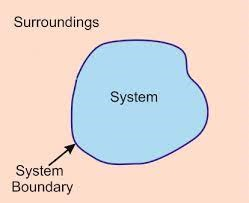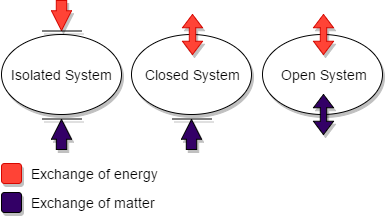# First Law of Thermodynamics Introduction, Principle, Types of Systems, Properties, Zeroth law of Thermodynamics ?

0

### First Law of Thermodynamics:

The word Thermodynamics means that the flow of heat. It deals with the energy changes accompanied with the physical and chemical processes. It deals with macroscopic properties (heat, work) and their inter relationships. The principles of thermodynamics are based on three laws of thermodynamics.

### System:

The system is the part of universe which is under thermodynamic consideration. It is separated from the rest of the universe by real or imaginary boundaries.

If the physical state of all constituents are in the same phase then it is called homogeneous system.

READ  Basics of green chemistry and day to day life..?

E.g., Mixture of gases.

If the physical state of the constituents are in different  phase then it is called heterogeneous system.

E.g., Mixture of oil and water.

### Surrounding:

The surface which is not a part of the system is called surroundings.

### Boundary:

The thing which separates the system from its surrounding is called boundary.### Types of systems:

Isolated system:

In this system, both energy and matter neither enter nor leave the system.

Closed system:

In this system energy is transferred to the surroundings but not the matter.

Open system:

The system can exchange both energy and matter with the surroundings.

READ  Second law of Thermodynamics and Various Types?### Properties of the system:

Extensive properties:

The property that depends on the mass or size of the system is called an extensive property.

E.g., Volume, Mass, Internal energy

Intensive properties:

The property that is independent of the mass or size of the system is called an intensive property.

E.g., Refractive index, density, temperature.

### First Law of Thermodynamics:

The first of thermodynamics is known as the law of conservation of energy, states that the total energy of an isolated system remains constant though it may change from one form to another.

### Other statements of First law of Thermodynamics:

• Whenever the energy of particular type disappears, an equivalent amount of another type must be produced.
• The total energy of a system and surrounding remains constant.
• Energy can neither be created nor destroyed, but may be converted from one form to another.
• The change in the internal energy of a closed system is equal to the energy that passes through its boundary as heat or work.
• Heat and work are two ways of changing a system’s internal energy.

### Internal Energy

• The internal energy of a system is an extensive property. It depends on the amount of the substances present in the system.
• The internal energy of a system is a state function. It depends only upon the state variables (T, P, V, n) of the system.
• The change in internal energy of a system is expressed as ∆U=Uf – Ui.
• In a cyclic process, there is no internal energy change. ∆Ucyclic=0.
READ  Calcium Carbonate Definition, Structure, Balancing Equation, Examples , Uses and Video ( study material ).

### Enthalpy

Enthalpy is a state function which depends entirely on the state functions, T, P and U. Enthalpy is usually expressed as the change in enthalpy for a process between initial and final states at constant pressure.

∆H= ∆U+ P∆V

READ  What is Raman Spectroscopy, Introduction, Principle, Types of scattering, Applications ?

### Standard heat of formation:

The standard heat of formation of a compound is defined as “the change in enthalpy that takes place when one mole of a compound is formed from its elements, present in their standard states”.

### Molar heat capacities:

When heat is supplied to a system, the molecules in the system absorb the heat and hence their kinetic energy increases, which in turn raises the temperature of the system from T1 to T2. This increase (T2 – T1) in temperature is directly proportional to the amount of heat absorbed and inversely proportional to mass of the substance.

READ  Magnesium and Magnesium Formula, Structure, Uses, Video.

q α m ∆T

q = c m ∆T

c = q / m ∆T

The constant c is called heat capacity.

c = q / m (T2 – T1)

### Joule – Thomson Effect

The phenomenon of change of temperature produced when a gas is made to expand adiabatically from a region of high pressure to a region of extremely low pressure is known as the Joule- Thomson effect.

### Inversion Temperature

The temperature below which a gas becomes cooler on expansion is known as inversion temperature.

### Zeroth law of Thermodynamics

If body A is in equilibrium with body C and body B is also in equilibrium with body C, then bodies A and B are in equilibrium with each other.